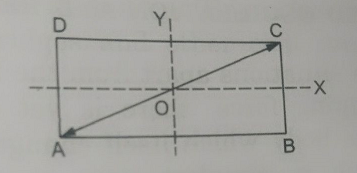Q

# Can someone explain - Oscillations and Waves - JEE Main-5

The composition of two simple harmonic motions of equal periods at right angle to each other and with a phase difference of  results in the displacement of the particle along

• Option 1)

Straight Line

• Option 2)

Circle

• Option 3)

Ellipse

• Option 4)

Figure of eight

118 Views

If  and

This is equation of straight line.

Resultant equation of two perpendicular SHM when δ = 0 -

Resultant equation

- wherein

It is a straight line with slopeOption 1)

Straight Line

This is correct.

Option 2)

Circle

This is incorrect.

Option 3)

Ellipse

This is incorrect.

Option 4)

Figure of eight

This is incorrect.

Exams
Articles
Questions